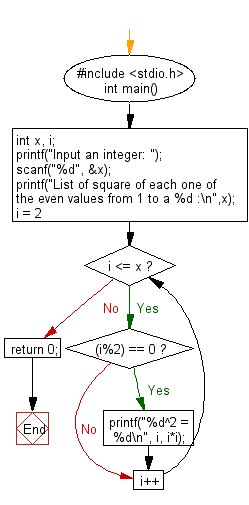﻿ C : Square of all even values from 1 to a specified value

# C Exercises: Print the square of each one of the even values from 1 to a specified value

## C Basic Declarations and Expressions: Exercise-30 with Solution

Write a C program to find and print the square of all the even values from 1 to a specified value.

C Code:

``````#include <stdio.h>
int main() {
int x, i; // Declare variables for user input and loop counter
printf("Input an integer: ");
scanf("%d", &x); // Prompt user for an integer

printf("List of square of each one of the even values from 1 to a %d :\n", x);

for(i = 2; i <= x; i++) { // Loop through numbers from 2 to x
if((i%2) == 0) { // Check if the number is even
printf("%d^2 = %d\n", i, i*i); // Print the square of the even number
}
}

return 0;
}
``````

Sample Output:

```List of square of each one of the even values from 1 to a 4 :
2^2 = 4
4^2 = 16
```

Flowchart:C Programming Code Editor:

What is the difficulty level of this exercise?

Test your Programming skills with w3resource's quiz.

﻿